Скачать презентацию Chapter 3 Working With Financial Statements Mc Graw-Hill Irwin

4f5524ea544b79bf5602afe25e39783d.ppt

• Количество слайдов: 34Chapter 3 Working With Financial Statements Mc. Graw-Hill/Irwin Copyright © 2010 by The Mc. Graw-Hill Companies, Inc. All rights reserved. 1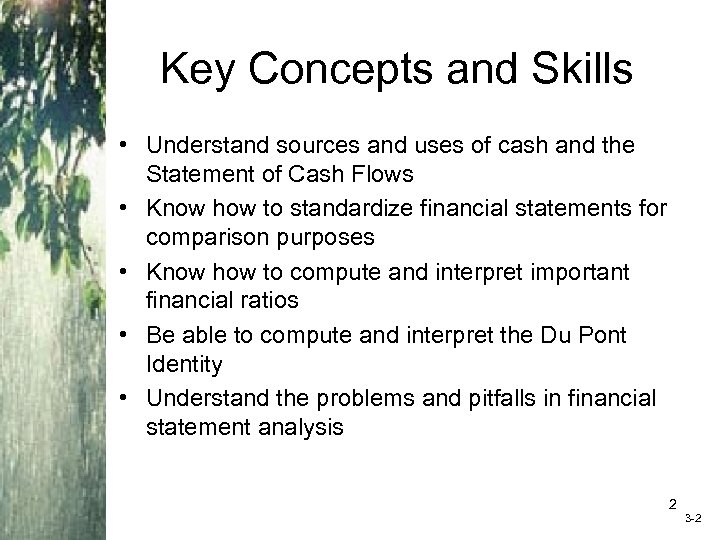Key Concepts and Skills • Understand sources and uses of cash and the Statement of Cash Flows • Know how to standardize financial statements for comparison purposes • Know how to compute and interpret important financial ratios • Be able to compute and interpret the Du Pont Identity • Understand the problems and pitfalls in financial statement analysis 2 3 -2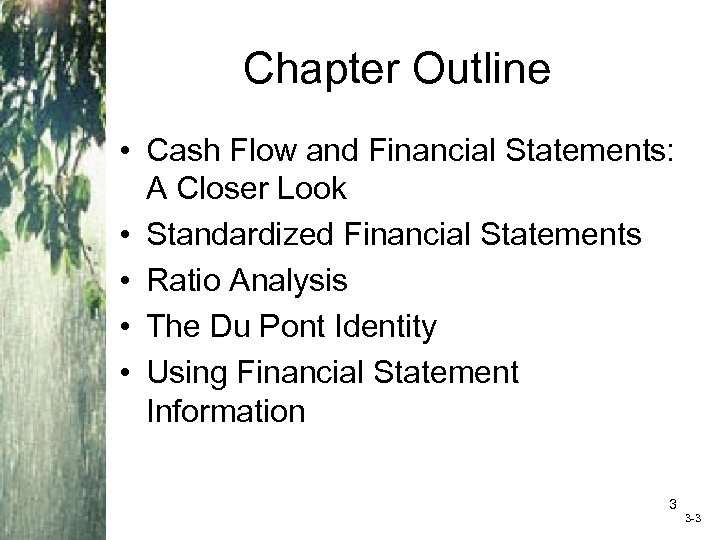Chapter Outline • Cash Flow and Financial Statements: A Closer Look • Standardized Financial Statements • Ratio Analysis • The Du Pont Identity • Using Financial Statement Information 3 3 -3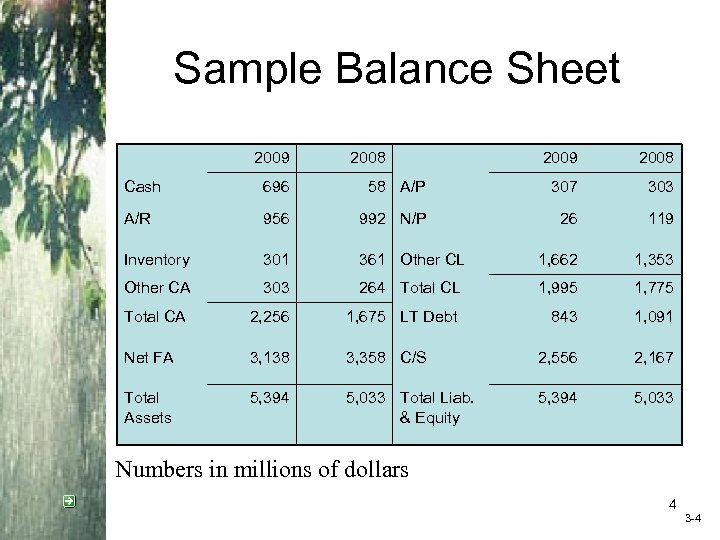Sample Balance Sheet 2009 2008 Cash 696 58 A/P 307 303 A/R 956 992 N/P 26 119 Inventory 301 361 Other CL 1, 662 1, 353 Other CA 303 264 Total CL 1, 995 1, 775 Total CA 2, 256 1, 675 LT Debt 843 1, 091 Net FA 3, 138 3, 358 C/S 2, 556 2, 167 Total Assets 5, 394 5, 033 Total Liab. & Equity 5, 394 5, 033 Numbers in millions of dollars 4 3 -4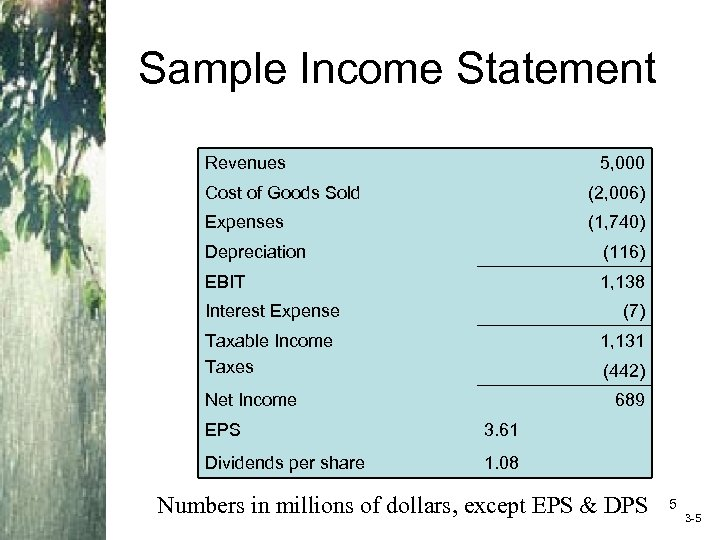Sample Income Statement Revenues 5, 000 Cost of Goods Sold (2, 006) Expenses (1, 740) Depreciation (116) EBIT 1, 138 Interest Expense (7) Taxable Income Taxes 1, 131 (442) Net Income 689 EPS 3. 61 Dividends per share 1. 08 Numbers in millions of dollars, except EPS & DPS 5 3 -5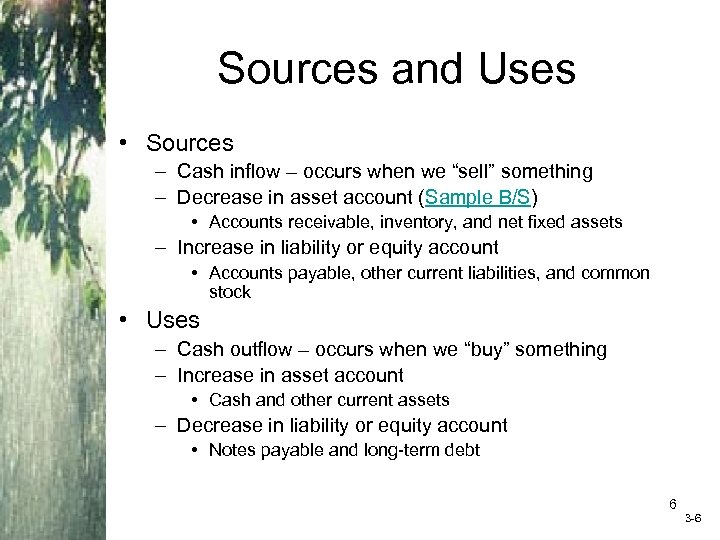Sources and Uses • Sources – Cash inflow – occurs when we “sell” something – Decrease in asset account (Sample B/S) • Accounts receivable, inventory, and net fixed assets – Increase in liability or equity account • Accounts payable, other current liabilities, and common stock • Uses – Cash outflow – occurs when we “buy” something – Increase in asset account • Cash and other current assets – Decrease in liability or equity account • Notes payable and long-term debt 6 3 -6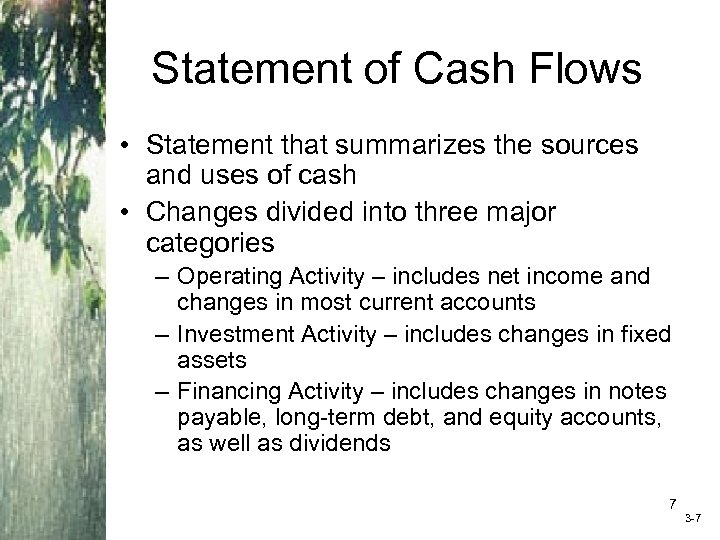Statement of Cash Flows • Statement that summarizes the sources and uses of cash • Changes divided into three major categories – Operating Activity – includes net income and changes in most current accounts – Investment Activity – includes changes in fixed assets – Financing Activity – includes changes in notes payable, long-term debt, and equity accounts, as well as dividends 7 3 -7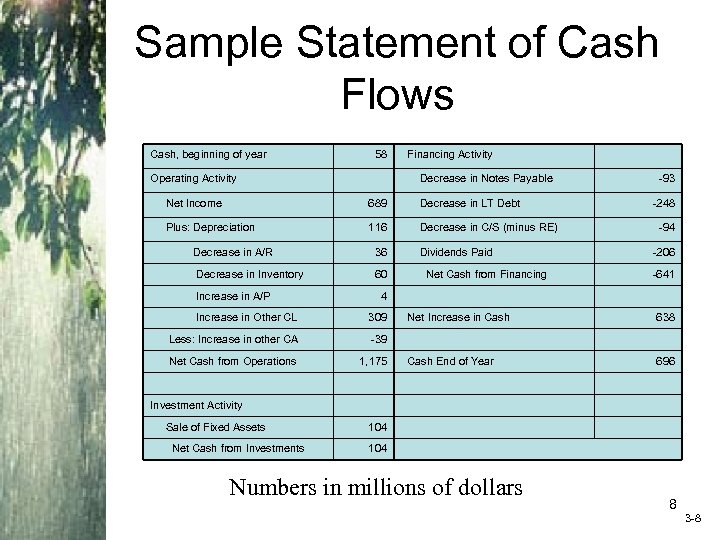Sample Statement of Cash Flows Cash, beginning of year 58 Operating Activity Financing Activity Decrease in Notes Payable Net Income 689 Decrease in LT Debt Plus: Depreciation 116 Decrease in C/S (minus RE) Decrease in A/R 36 Decrease in Inventory 60 Increase in A/P Dividends Paid Net Cash from Financing -93 -248 -94 -206 -641 4 Increase in Other CL 309 Less: Increase in other CA -39 Net Cash from Operations 1, 175 Net Increase in Cash 638 Cash End of Year 696 Investment Activity Sale of Fixed Assets Net Cash from Investments 104 Numbers in millions of dollars 8 3 -8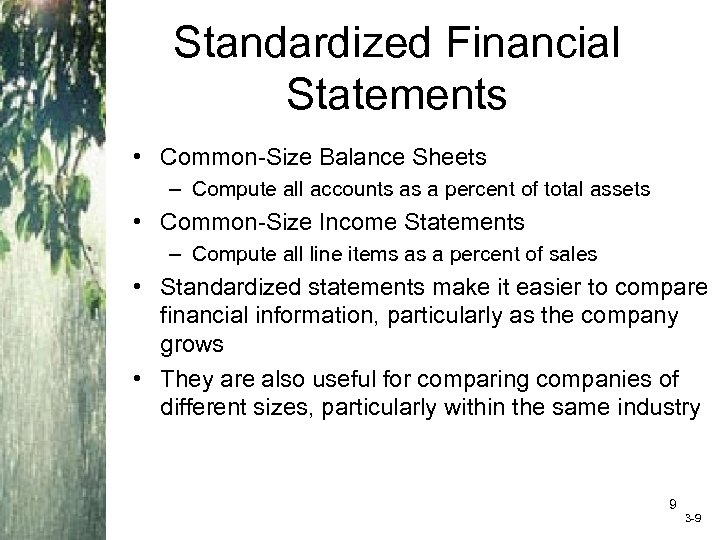Standardized Financial Statements • Common-Size Balance Sheets – Compute all accounts as a percent of total assets • Common-Size Income Statements – Compute all line items as a percent of sales • Standardized statements make it easier to compare financial information, particularly as the company grows • They are also useful for comparing companies of different sizes, particularly within the same industry 9 3 -9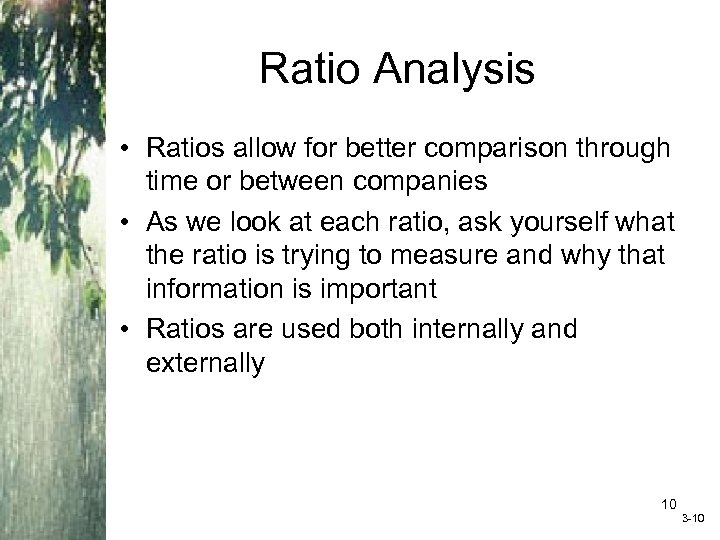Ratio Analysis • Ratios allow for better comparison through time or between companies • As we look at each ratio, ask yourself what the ratio is trying to measure and why that information is important • Ratios are used both internally and externally 10 3 -10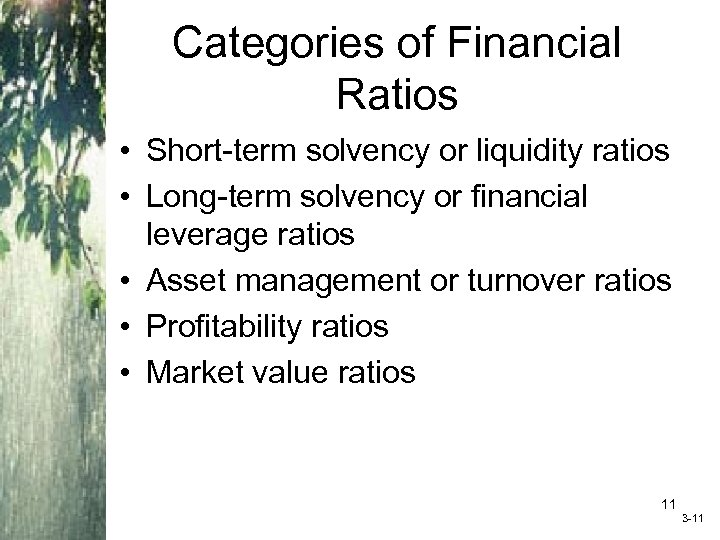Categories of Financial Ratios • Short-term solvency or liquidity ratios • Long-term solvency or financial leverage ratios • Asset management or turnover ratios • Profitability ratios • Market value ratios 11 3 -11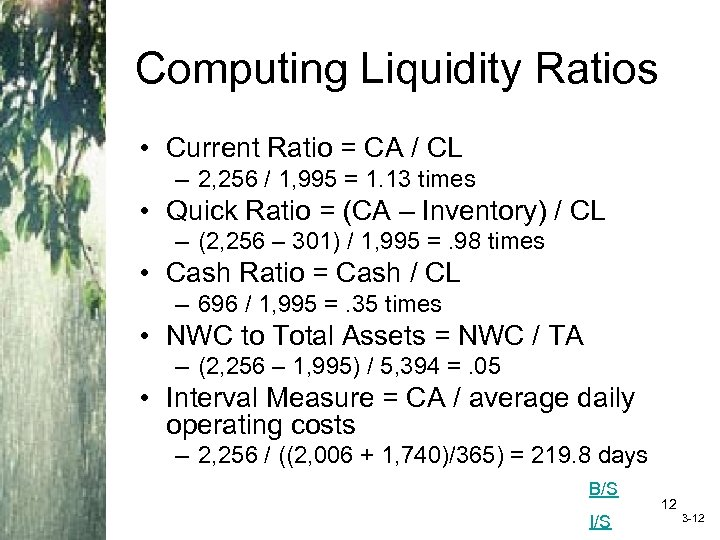Computing Liquidity Ratios • Current Ratio = CA / CL – 2, 256 / 1, 995 = 1. 13 times • Quick Ratio = (CA – Inventory) / CL – (2, 256 – 301) / 1, 995 =. 98 times • Cash Ratio = Cash / CL – 696 / 1, 995 =. 35 times • NWC to Total Assets = NWC / TA – (2, 256 – 1, 995) / 5, 394 =. 05 • Interval Measure = CA / average daily operating costs – 2, 256 / ((2, 006 + 1, 740)/365) = 219. 8 days B/S I/S 12 3 -12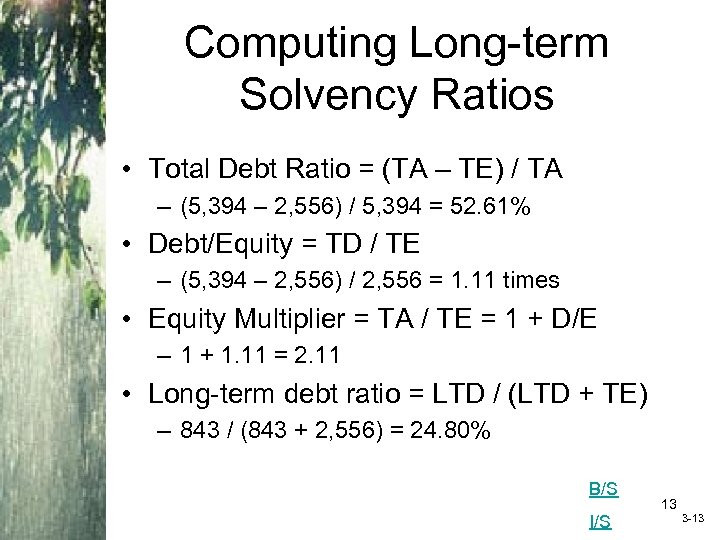Computing Long-term Solvency Ratios • Total Debt Ratio = (TA – TE) / TA – (5, 394 – 2, 556) / 5, 394 = 52. 61% • Debt/Equity = TD / TE – (5, 394 – 2, 556) / 2, 556 = 1. 11 times • Equity Multiplier = TA / TE = 1 + D/E – 1 + 1. 11 = 2. 11 • Long-term debt ratio = LTD / (LTD + TE) – 843 / (843 + 2, 556) = 24. 80% B/S I/S 13 3 -13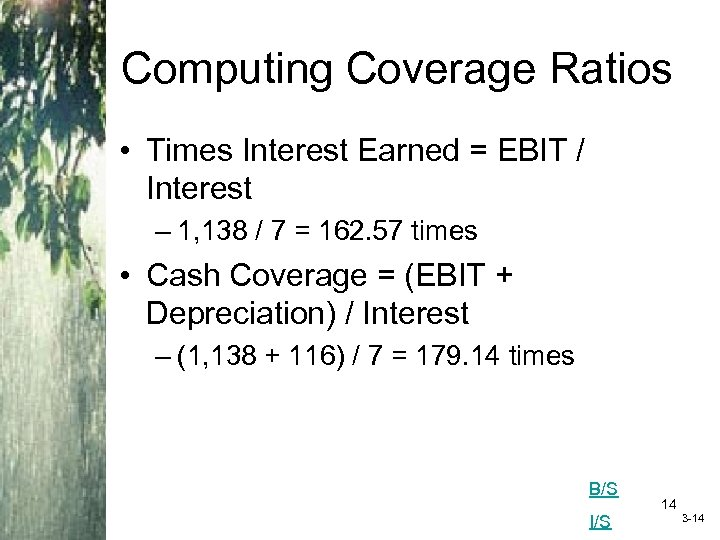Computing Coverage Ratios • Times Interest Earned = EBIT / Interest – 1, 138 / 7 = 162. 57 times • Cash Coverage = (EBIT + Depreciation) / Interest – (1, 138 + 116) / 7 = 179. 14 times B/S I/S 14 3 -14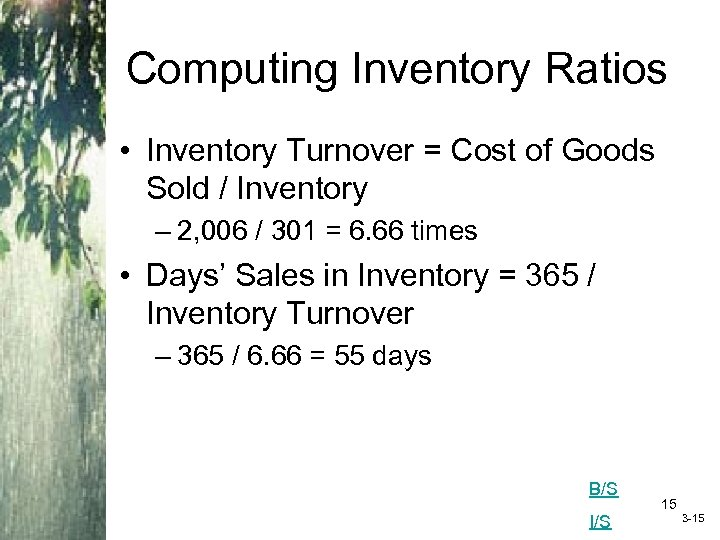Computing Inventory Ratios • Inventory Turnover = Cost of Goods Sold / Inventory – 2, 006 / 301 = 6. 66 times • Days’ Sales in Inventory = 365 / Inventory Turnover – 365 / 6. 66 = 55 days B/S I/S 15 3 -15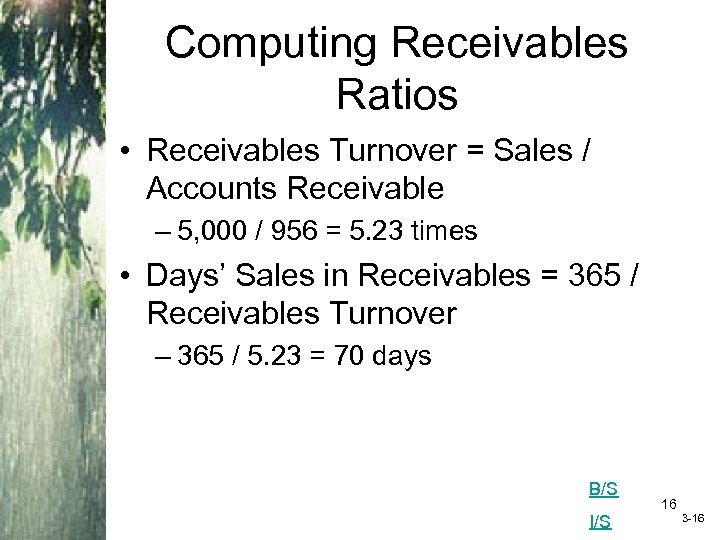Computing Receivables Ratios • Receivables Turnover = Sales / Accounts Receivable – 5, 000 / 956 = 5. 23 times • Days’ Sales in Receivables = 365 / Receivables Turnover – 365 / 5. 23 = 70 days B/S I/S 16 3 -16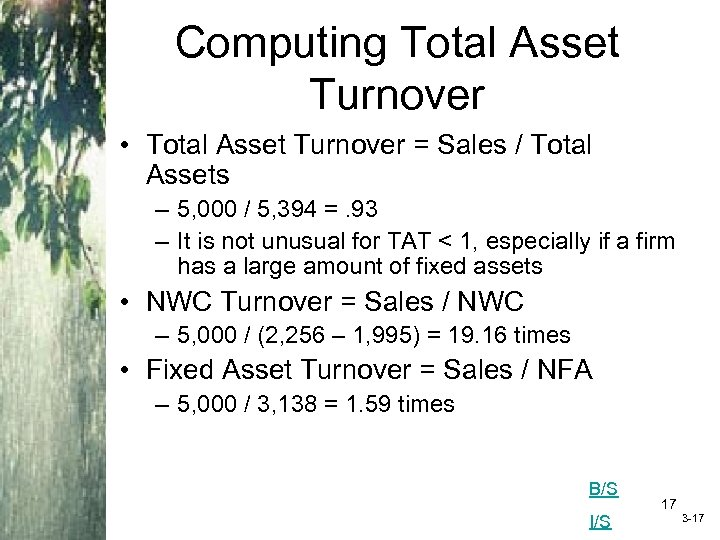Computing Total Asset Turnover • Total Asset Turnover = Sales / Total Assets – 5, 000 / 5, 394 =. 93 – It is not unusual for TAT < 1, especially if a firm has a large amount of fixed assets • NWC Turnover = Sales / NWC – 5, 000 / (2, 256 – 1, 995) = 19. 16 times • Fixed Asset Turnover = Sales / NFA – 5, 000 / 3, 138 = 1. 59 times B/S I/S 17 3 -17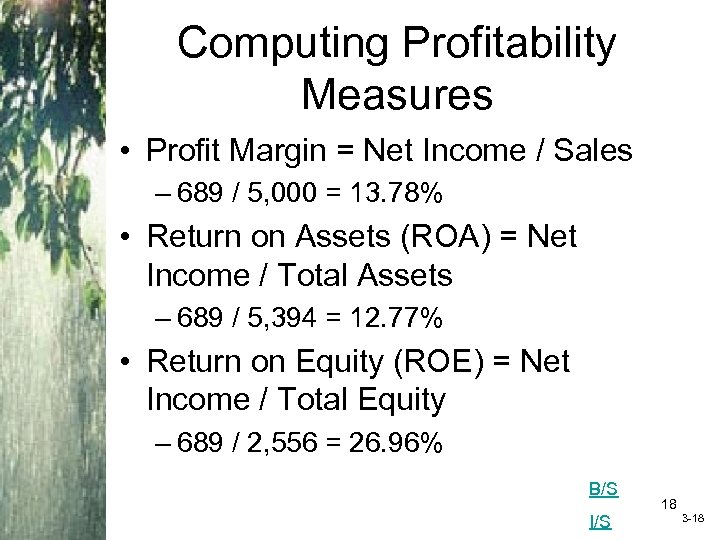Computing Profitability Measures • Profit Margin = Net Income / Sales – 689 / 5, 000 = 13. 78% • Return on Assets (ROA) = Net Income / Total Assets – 689 / 5, 394 = 12. 77% • Return on Equity (ROE) = Net Income / Total Equity – 689 / 2, 556 = 26. 96% B/S I/S 18 3 -18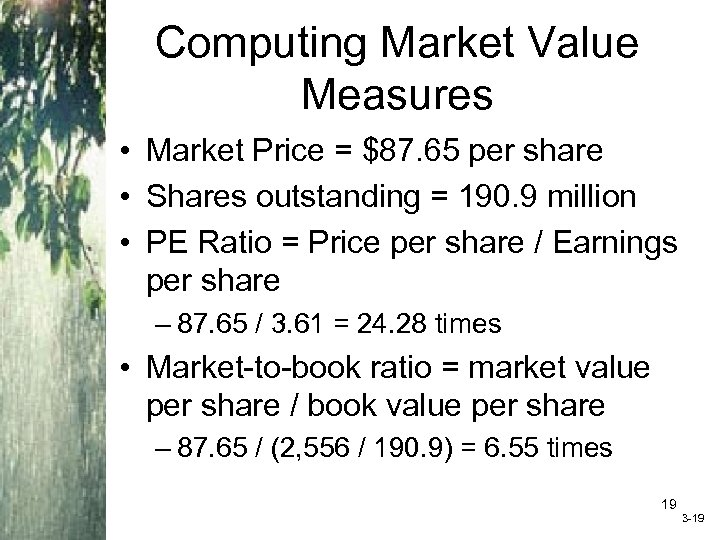Computing Market Value Measures • Market Price = \$87. 65 per share • Shares outstanding = 190. 9 million • PE Ratio = Price per share / Earnings per share – 87. 65 / 3. 61 = 24. 28 times • Market-to-book ratio = market value per share / book value per share – 87. 65 / (2, 556 / 190. 9) = 6. 55 times 19 3 -19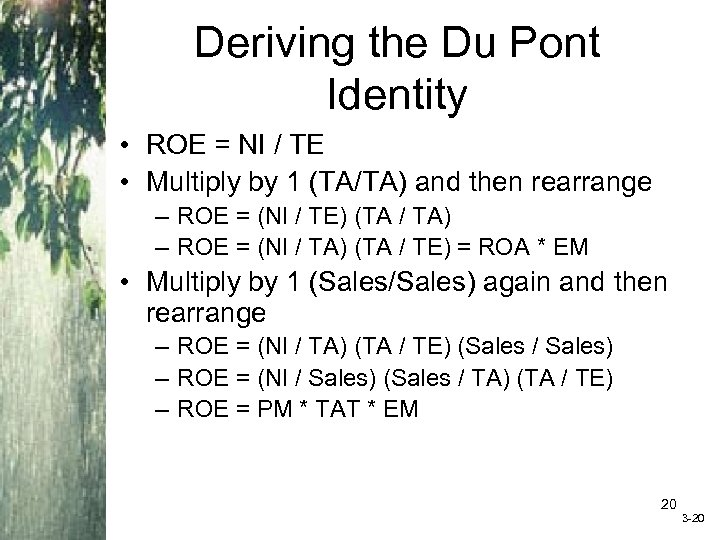Deriving the Du Pont Identity • ROE = NI / TE • Multiply by 1 (TA/TA) and then rearrange – ROE = (NI / TE) (TA / TA) – ROE = (NI / TA) (TA / TE) = ROA * EM • Multiply by 1 (Sales/Sales) again and then rearrange – ROE = (NI / TA) (TA / TE) (Sales / Sales) – ROE = (NI / Sales) (Sales / TA) (TA / TE) – ROE = PM * TAT * EM 20 3 -20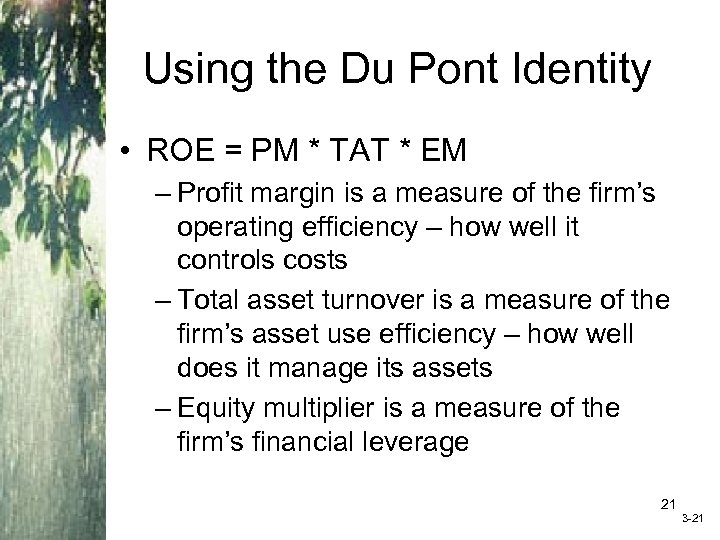Using the Du Pont Identity • ROE = PM * TAT * EM – Profit margin is a measure of the firm’s operating efficiency – how well it controls costs – Total asset turnover is a measure of the firm’s asset use efficiency – how well does it manage its assets – Equity multiplier is a measure of the firm’s financial leverage 21 3 -21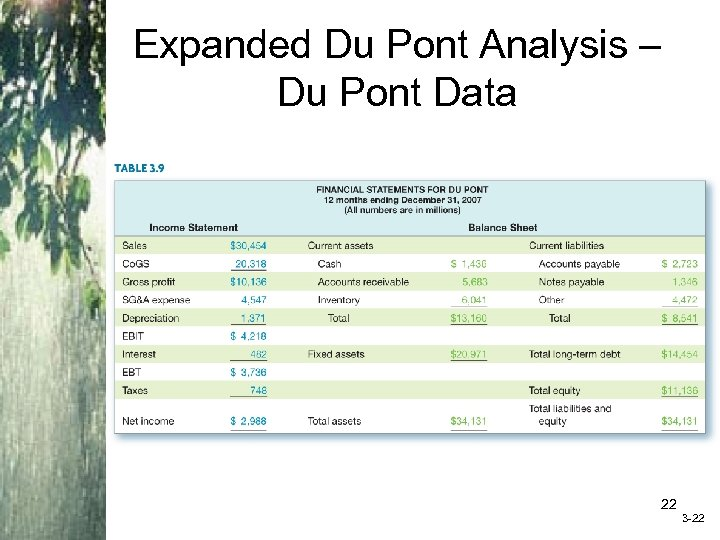Expanded Du Pont Analysis – Du Pont Data 22 3 -22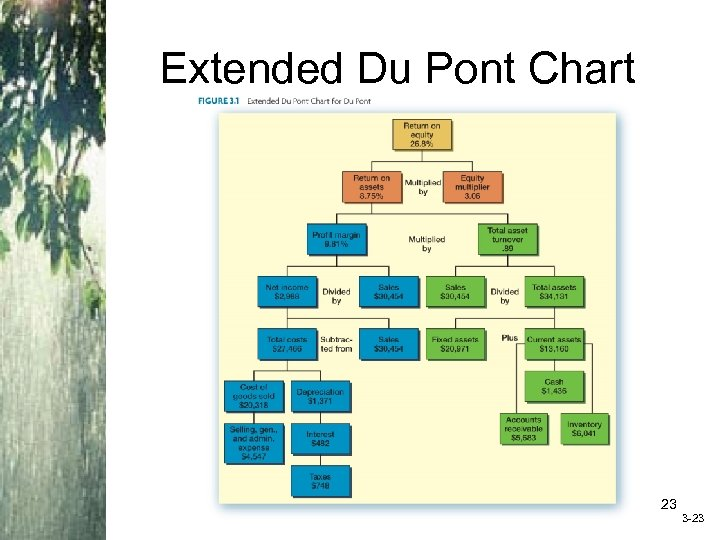Extended Du Pont Chart Insert Figure 3. 1 (Extended Du. Pont Chart) 23 3 -23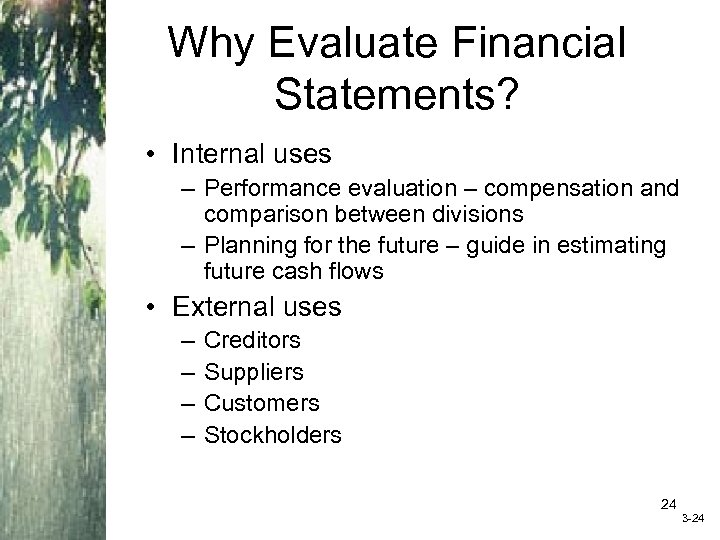Why Evaluate Financial Statements? • Internal uses – Performance evaluation – compensation and comparison between divisions – Planning for the future – guide in estimating future cash flows • External uses – – Creditors Suppliers Customers Stockholders 24 3 -24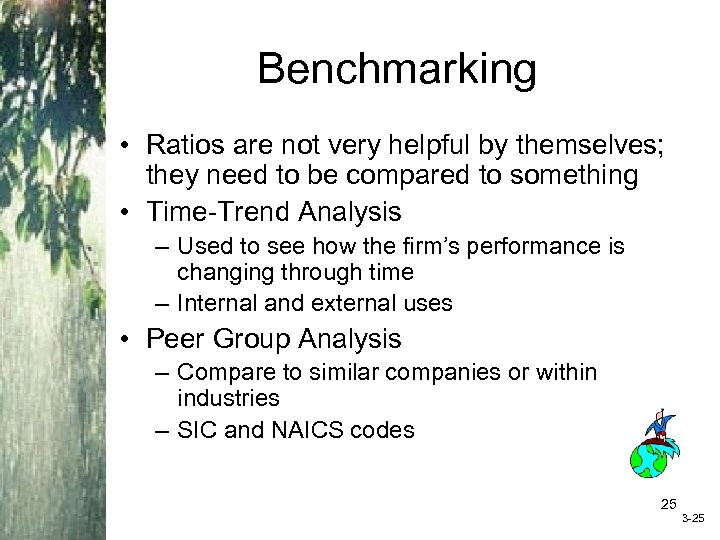Benchmarking • Ratios are not very helpful by themselves; they need to be compared to something • Time-Trend Analysis – Used to see how the firm’s performance is changing through time – Internal and external uses • Peer Group Analysis – Compare to similar companies or within industries – SIC and NAICS codes 25 3 -25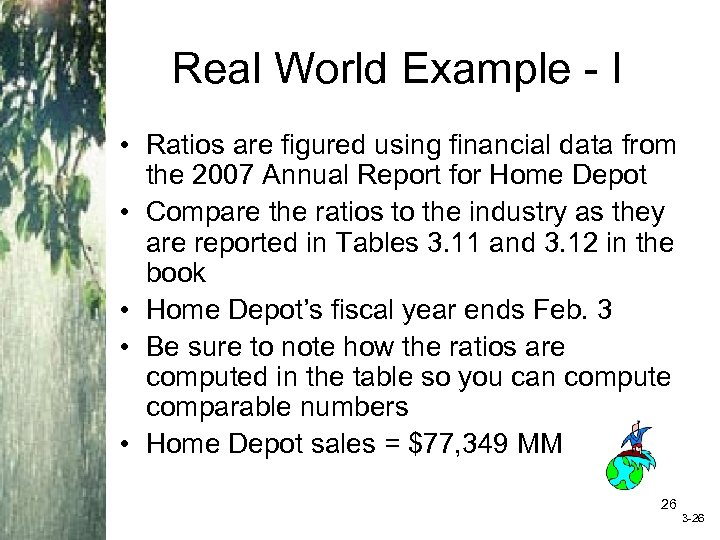Real World Example - I • Ratios are figured using financial data from the 2007 Annual Report for Home Depot • Compare the ratios to the industry as they are reported in Tables 3. 11 and 3. 12 in the book • Home Depot’s fiscal year ends Feb. 3 • Be sure to note how the ratios are computed in the table so you can compute comparable numbers • Home Depot sales = \$77, 349 MM 26 3 -26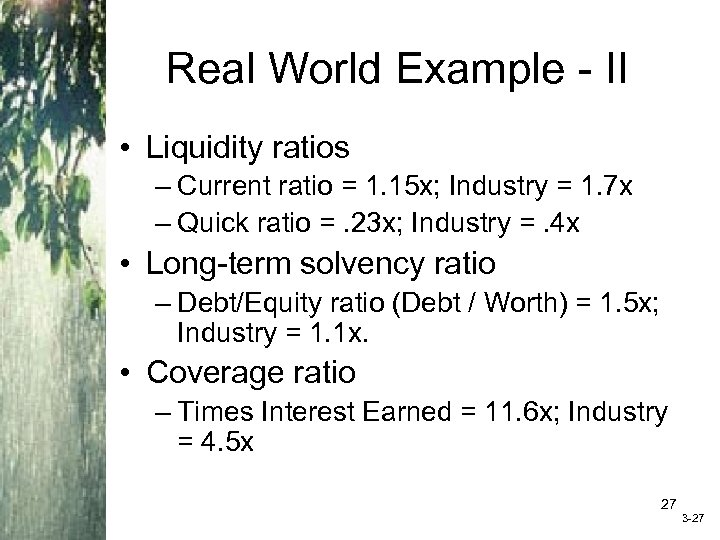Real World Example - II • Liquidity ratios – Current ratio = 1. 15 x; Industry = 1. 7 x – Quick ratio =. 23 x; Industry =. 4 x • Long-term solvency ratio – Debt/Equity ratio (Debt / Worth) = 1. 5 x; Industry = 1. 1 x. • Coverage ratio – Times Interest Earned = 11. 6 x; Industry = 4. 5 x 27 3 -27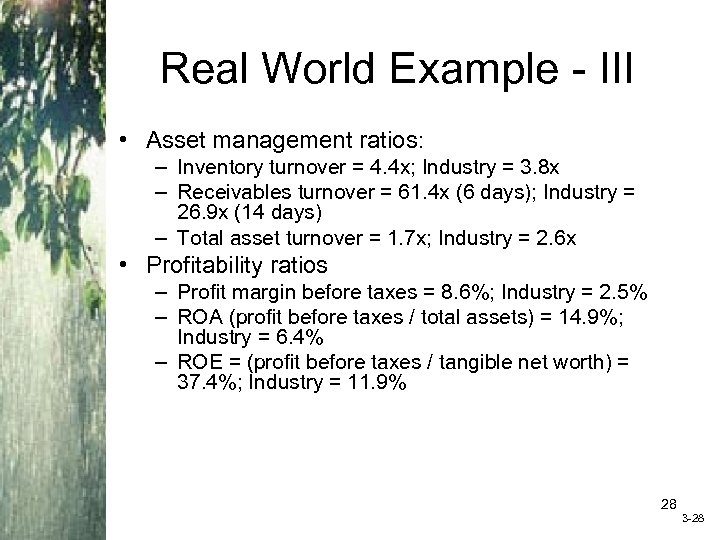Real World Example - III • Asset management ratios: – Inventory turnover = 4. 4 x; Industry = 3. 8 x – Receivables turnover = 61. 4 x (6 days); Industry = 26. 9 x (14 days) – Total asset turnover = 1. 7 x; Industry = 2. 6 x • Profitability ratios – Profit margin before taxes = 8. 6%; Industry = 2. 5% – ROA (profit before taxes / total assets) = 14. 9%; Industry = 6. 4% – ROE = (profit before taxes / tangible net worth) = 37. 4%; Industry = 11. 9% 28 3 -28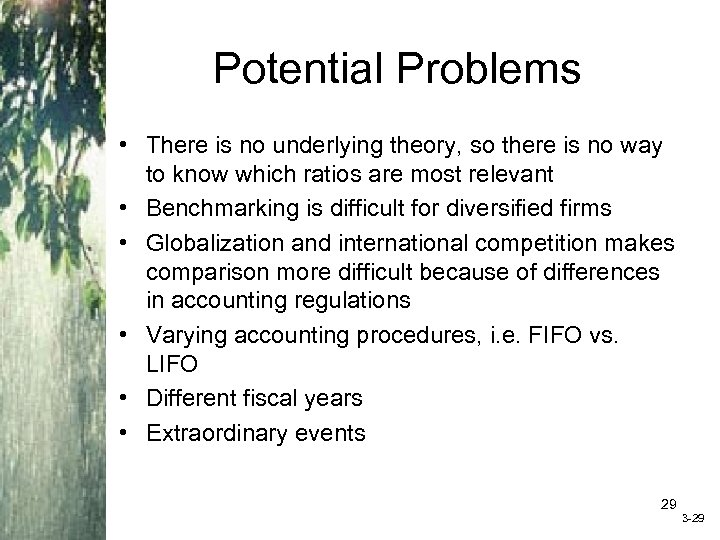Potential Problems • There is no underlying theory, so there is no way to know which ratios are most relevant • Benchmarking is difficult for diversified firms • Globalization and international competition makes comparison more difficult because of differences in accounting regulations • Varying accounting procedures, i. e. FIFO vs. LIFO • Different fiscal years • Extraordinary events 29 3 -29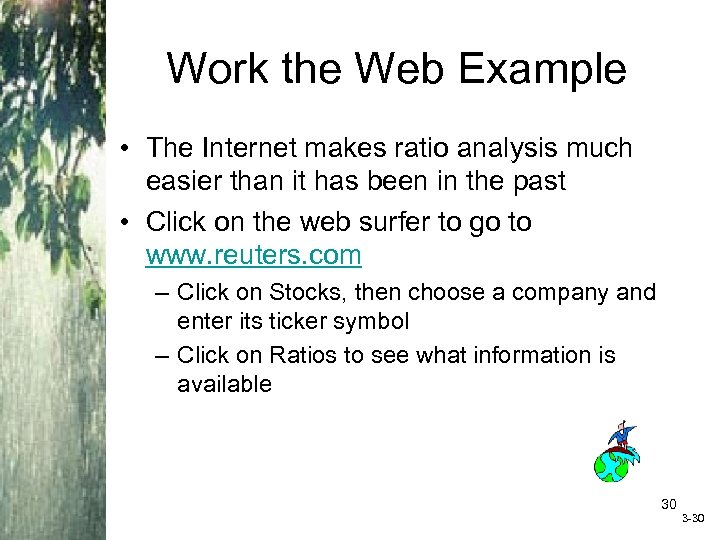Work the Web Example • The Internet makes ratio analysis much easier than it has been in the past • Click on the web surfer to go to www. reuters. com – Click on Stocks, then choose a company and enter its ticker symbol – Click on Ratios to see what information is available 30 3 -30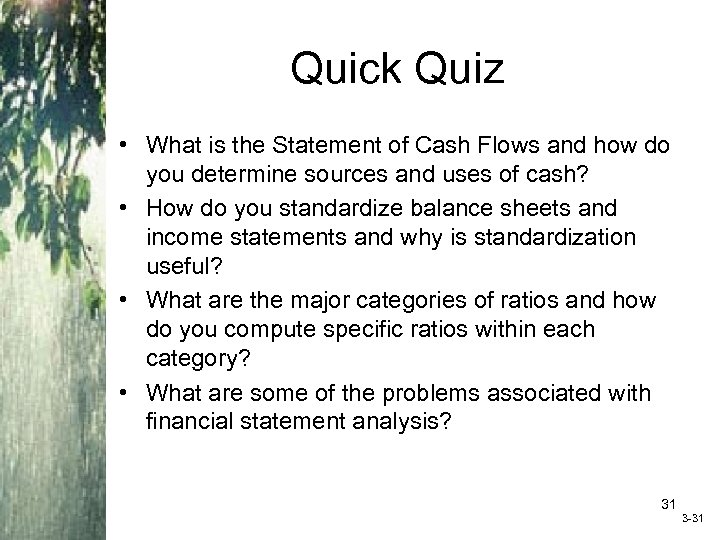Quick Quiz • What is the Statement of Cash Flows and how do you determine sources and uses of cash? • How do you standardize balance sheets and income statements and why is standardization useful? • What are the major categories of ratios and how do you compute specific ratios within each category? • What are some of the problems associated with financial statement analysis? 31 3 -31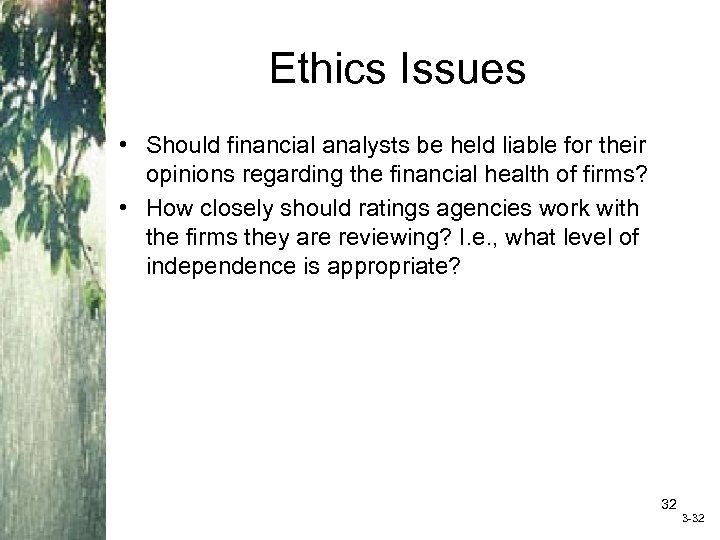Ethics Issues • Should financial analysts be held liable for their opinions regarding the financial health of firms? • How closely should ratings agencies work with the firms they are reviewing? I. e. , what level of independence is appropriate? 32 3 -32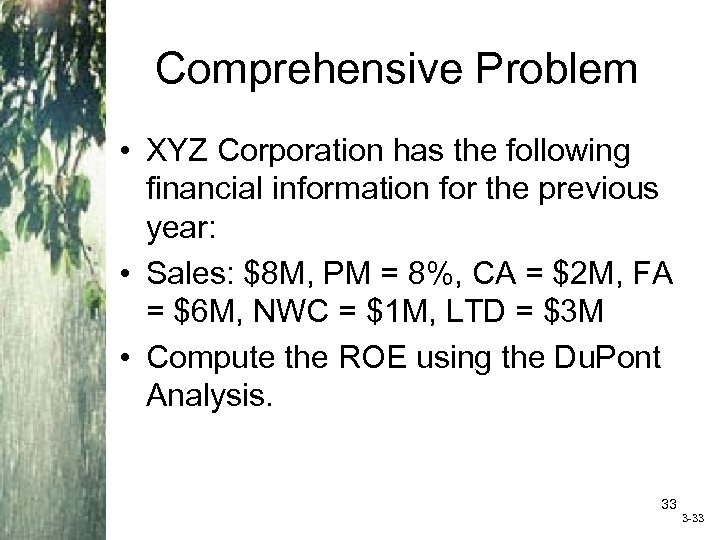Comprehensive Problem • XYZ Corporation has the following financial information for the previous year: • Sales: \$8 M, PM = 8%, CA = \$2 M, FA = \$6 M, NWC = \$1 M, LTD = \$3 M • Compute the ROE using the Du. Pont Analysis. 33 3 -33End of Chapter 34 3 -34